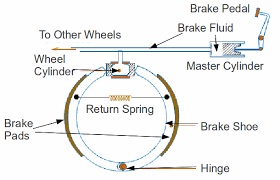## Posts

Showing posts from January, 2018

### Q. Explain Principle, Purpose and Features of Nuclear ReactorsNUCLEAR REACTOR
DEFINITION: Nuclear reactor is a device which is used to perform controlled fission reaction and extract the hidden energy from the nucleus.

PURPOSE: Following are some main purpose of the nuclear reactor.
1- To convert controlled fission reaction to controlled chain reaction.
2- To produce large amount of energy.
3- To produce radioisotopes.

PRINCIPLE: It is used on the principle of nuclear fission a process in which heavy nucleus breaks in to lighter nuclei with the emission of large amount of energy”.

FEATURES OF NUCLEAR REACTOR: Following are the general features, which are common or almost nuclear reactor.

1. NUCLEAR FUEL: A Material consisting of the fissionable isotopes is called reactor fuel that may be used in a reactor. For example (Uranium 92U235).

2. MODERATORS: In the nuclear fission process at least one or more neutrons are produce per fission. To reduce the energy of neutron some suitable material is required which are known as moderators. The ordinary …

### How Hydraulic Brakes work?The working of hydraulic brakes is based on the principle of Pascal law. These brakes are used in automobiles. It consists of a tube which contains oil called brake oil. One end of the tube is fitted with a piston working in a master cylinder. There are in all four tubes through which the master cylinder is joined to four small cylinders (each containing a piston of large area) one for each wheel of the automobile. These small cylinders are called brake cylinders. When the brake pedal is pressed by applying a force, the piston in the master cylinder begins to move inside the tube and exerts pressure on the oil. This  pressure is transmitted to the oil in the brake cylinder. The undiminished transmitted pressure pushes the piston in brake cylinder which acts as a brake shoe attached to a caliper. The resulting friction stops the wheel. When the pressure on the pedal is released, the spring which connects the two brake-shoes contracts and pulls them off from the rotor. Thus the wheel i…

### WORK-ENERGY RELATION

WORK ENERGY EQUATION
DERIVATION: Let us consider a body of mass “m” is placed at point A at a height h from the surface of earth.At this point the body possesses gravitational potential energy equal to mgh w.r.t point C lying on the ground.             Now consider a point B at a distance x below the point A during downward motion of body.At this stage the height of the body becomes (h-x).
so, potential energy at point B becomes,                                                 P.E=mg(h-x) As we know that potential energy at point B is less than the potential energy at point A,i.e.                                                 mg(h-x) < mgh             or                                 mgh – mgx <mgh
The loss in potential energy at point B is mgx. The Kinetic Energy at point A is equal to zero because the body is at rest.During its downward motion its velocity increases ,so its kinetic energy also increases.If there is no air friction then the loss of P.E is equal to the ga…